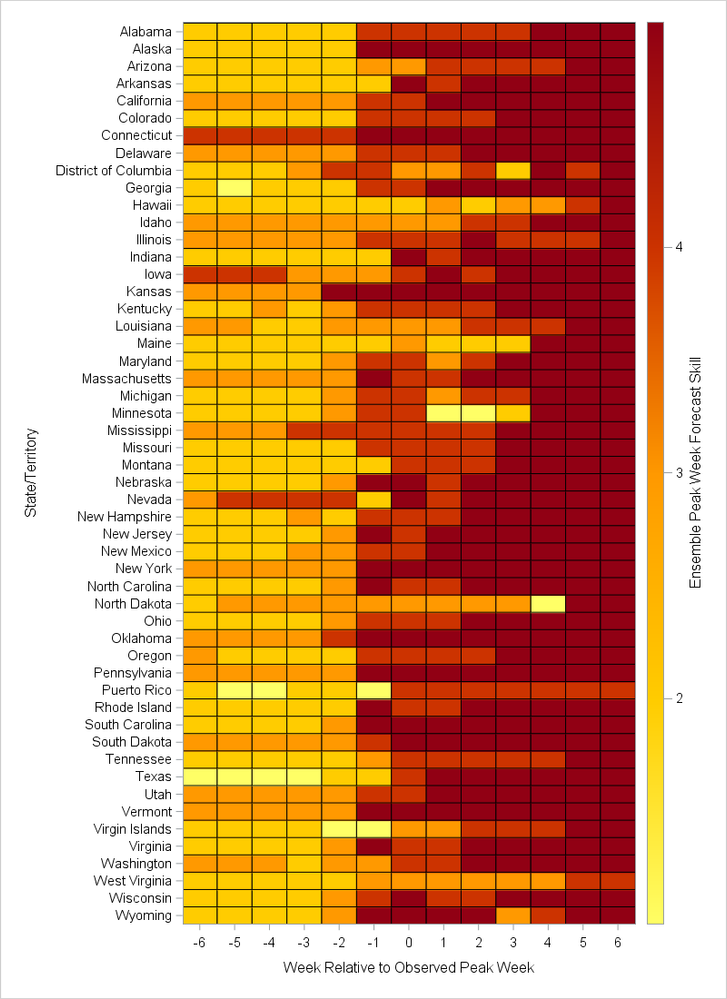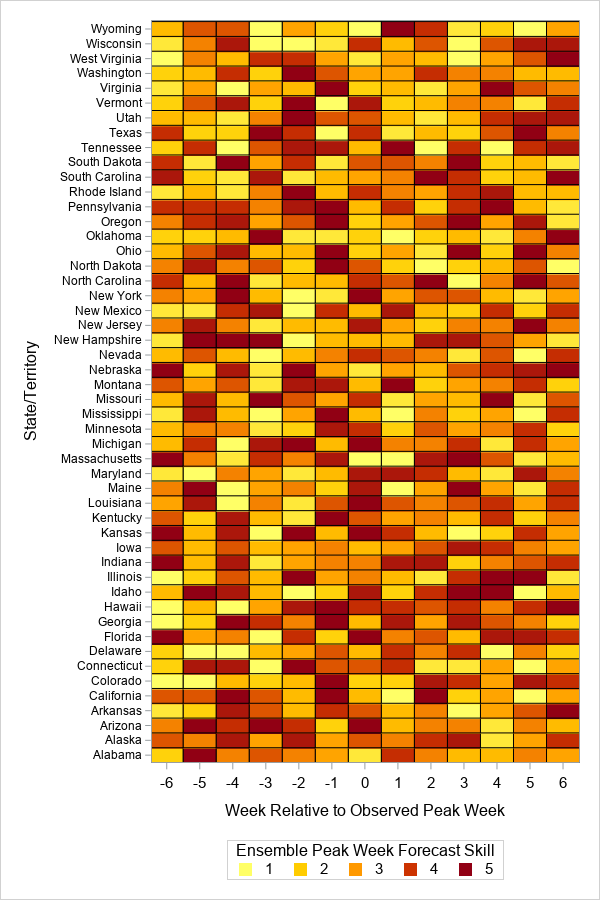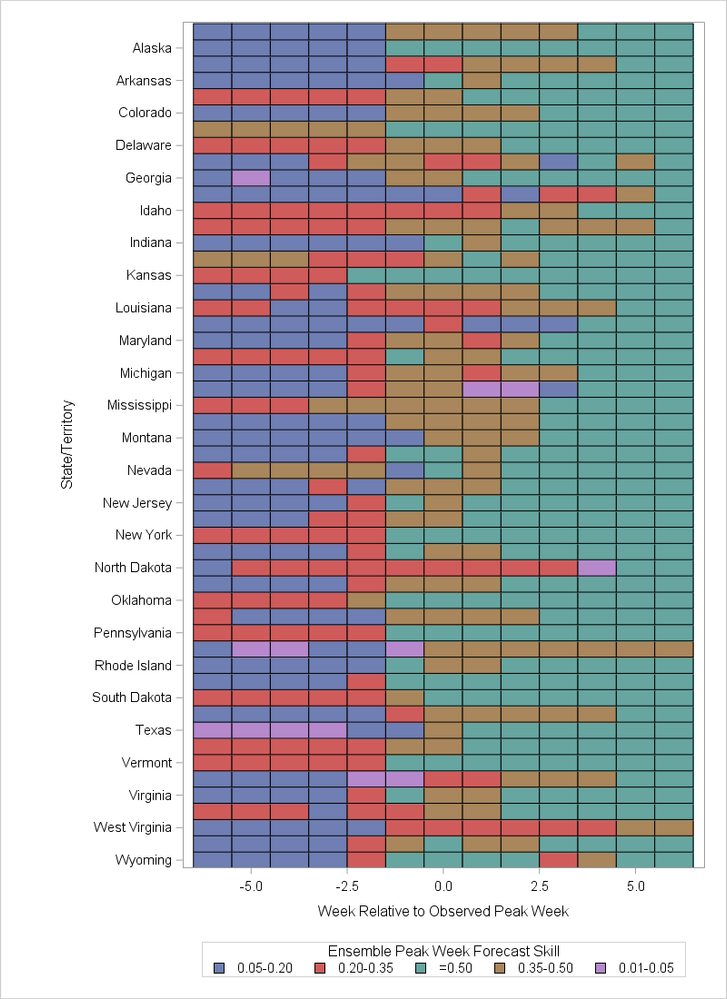## Discrete legend for proc sgplot heat map

Hello all and thank you in advance,

I have the below code to produce a heat map. My color response variable is discrete, with values from 1-5. Obviously the "gradlegend" creates the gradient legend, but I don't know what to replace it with to get a discrete legend. As is, the gradient legend both hides my minimum and maximum values for display, and the gradient just doesn't really make sense when the values can only be one of five colors. I tried "discretelegend", which didn't work, and this thread https://communities.sas.com/t5/Graphics-Programming/Please-explainh-the-illogical-gradlegend-in-foll..., which also wasn't the ticket. Does anyone know how to get the legend to display my discrete values? Thank you in advance!

``````proc sgplot data=heatmap;
heatmap x=relative_wk y=territory / discretex colormodel=(cxFFFF66 cxFFCC00 cxFF9900 cxCC3300 /*cx800000*/ cx910014) colorresponse=Skill_bin
outline;
gradlegend  / title="Ensemble Peak Week Forecast Skill";
xaxis label="Week Relative to Observed Peak Week";
yaxis label="State/Territory";
format Skill_bin b.;
run;``````1 ACCEPTED SOLUTION

Accepted Solutions

## Re: Discrete legend for proc sgplot heat map

1. Delete your COLORMODEL= option. That only applies to a continuous color response variable.

2. Add DISCRETEX option to the HEATMAPPARM statement.

3. If you use the XAXIS statement, you also need to add TYPE=DISCRETE to that statement.

``````data heatmap;
set sashelp.us_data (where=(statecode not in ('DC' 'PR')));
territory=statename;
do relative_wk = -6 to 6 by 1;
Skill_bin = rand("Integer", 1, 5);
output;
end;
run;

title;
ods graphics / height=800px width=480px;
proc sgplot data=heatmap;
heatmapparm x=relative_wk y=territory colorgroup=Skill_bin /
outline discretex;
keylegend  / title="Ensemble Peak Week Forecast Skill";
xaxis type=discrete label="Week Relative to Observed Peak Week";
yaxis reverse label="State/Territory";
run;``````

9 REPLIES 9Ksharp
Super User

## Re: Discrete legend for proc sgplot heat map

Do you have IML ?

This example is taken from iml documentation.

``````proc iml;
use Sashelp.Cars;
read all var _NUM_ into Y[c=varNames];
close Sashelp.Cars;
corr = corr(Y);
Bins = {"1: V. Neg", "2: Neg", "3: Neutral", "4: Pos", "5: V. Pos"};
idx = bin(corr, {-1, -0.6, -0.2, 0.2, 0.6, 1});
disCorr = shape(Bins[idx], nrow(corr));

call HeatmapDisc(disCorr) title="Correlations"
xvalues=varNames yvalues=varNames
LegendTitle="Magnitude";
quit;``````

## Re: Discrete legend for proc sgplot heat map

If you've got a fairly recent version of SAS, one way to do it would be to use the new 'legenditem' statement to custom-create each item you want in the legend ...

data heatmap; set sashelp.us_data (where=(statecode not in ('DC' 'PR')));
territory=statename;
do relative_wk = -6 to 6 by 1;
Skill_bin = rand("Integer", 1, 10);
output;
end;
run;

ods graphics / width=600px height=900px;
proc sgplot data=heatmap;
heatmap x=relative_wk y=territory / discretex
colormodel=(cxFFFF66 cxFFCC00 cxFF9900 cxCC3300 cx910014)
colorresponse=Skill_bin outline name='heat';

legenditem type=marker name='one' / label='1' markerattrs=(symbol=squarefilled size=9pt color=cxFFFF66);
legenditem type=marker name='two' / label='2' markerattrs=(symbol=squarefilled size=9pt color=cxFFCC00);
legenditem type=marker name='three' / label='3' markerattrs=(symbol=squarefilled size=9pt color=cxFF9900);
legenditem type=marker name='four' / label='4' markerattrs=(symbol=squarefilled size=9pt color=cxCC3300);
legenditem type=marker name='five' / label='5' markerattrs=(symbol=squarefilled size=9pt color=cx910014);

keylegend 'one' 'two' 'three' 'four' 'five' / title="Ensemble Peak Week Forecast Skill";

xaxis label="Week Relative to Observed Peak Week";
yaxis label="State/Territory" valueattrs=(size=8pt);
run;## Re: Discrete legend for proc sgplot heat map

I would switch to the HEATMAPPARM statement with the COLORGROUP= option. You can then use the DISCRETELEGEND statement to get the legend. For an example of syntax, see the last code segment at

https://blogs.sas.com/content/iml/2017/04/26/visualize-design-matrix.html

This assumes that you have one observation per cell. That is, the data are pre-summarized into bins.

## Re: Discrete legend for proc sgplot heat map

Thank you. The HEATMAPPARM statement worked halfway. Using the below code, I now get my discrete legend (yay!). However, my XAXIS is no longer discrete (i.e., instead of -6 to 6 by 1, I get -5 to 5 by 2.5), and any statement I add to fix that doesn't work...

``````proc sgplot data=heatmap;
heatmapparm x=relative_wk y=territory colorgroup=Skill_bin /
colormodel=(cxFFFF66 cxFFCC00 cxFF9900 cxCC3300 cx910014)
outline;
discretelegend  / title="Ensemble Peak Week Forecast Skill";
xaxis label="Week Relative to Observed Peak Week";
yaxis label="State/Territory";
format Skill_bin b.;
run;``````

I had "discretex" in the layout statement after the slash and before the outline argument. I received this error

`WARNING: HEATMAPPARM statement has a conflict with the axis type. The plot will not be drawn.  `

So, as per SAS support (http://support.sas.com/kb/55/717.html), I changed it to "Discretex=true" and just got a syntax error/nothing ran.So, in summary, the above legend is what I want, but the x axis isn't right anymore. Any idea how I can get my original x axis back? Thank you!!

## Re: Discrete legend for proc sgplot heat map

1. Delete your COLORMODEL= option. That only applies to a continuous color response variable.

2. Add DISCRETEX option to the HEATMAPPARM statement.

3. If you use the XAXIS statement, you also need to add TYPE=DISCRETE to that statement.

``````data heatmap;
set sashelp.us_data (where=(statecode not in ('DC' 'PR')));
territory=statename;
do relative_wk = -6 to 6 by 1;
Skill_bin = rand("Integer", 1, 5);
output;
end;
run;

title;
ods graphics / height=800px width=480px;
proc sgplot data=heatmap;
heatmapparm x=relative_wk y=territory colorgroup=Skill_bin /
outline discretex;
keylegend  / title="Ensemble Peak Week Forecast Skill";
xaxis type=discrete label="Week Relative to Observed Peak Week";
yaxis reverse label="State/Territory";
run;``````

## Re: Discrete legend for proc sgplot heat map

Thank you very much!Ksharp
Super User

## Re: Discrete legend for proc sgplot heat mapKsharp
Super User

## Re: Discrete legend for proc sgplot heat map

For future reference, I wrote a blog post that describes how to use PROC SGPLOT to create a discrete heatmap with a discrete legend:

Create a discrete heat map with PROC SGPLOT

Discussion stats
• 9 replies
• 2858 views
• 8 likes
• 4 in conversation